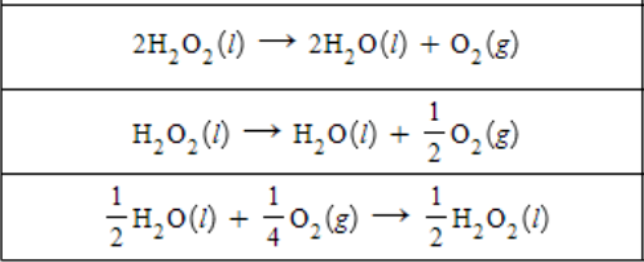🤓 Based on our data, we think this question is relevant for Professor Toole's class at UTOLEDO.

# Solution: A chemist measures the enthalpy change ΔH during the following reaction: 2H2O (l) + O2 (g) → 2H2O2 (l)            ΔH = 196. kJ Use this information to calculate ΔH of each reaction. Round each of your answers to the nearest kJ/mol.

###### Problem

A chemist measures the enthalpy change ΔH during the following reaction:

2H2O (l) + O2 (g) → 2H2O2 (l)            ΔH = 196. kJ

Use this information to calculate ΔH of each reaction. Round each of your answers to the nearest kJ/mol.###### Solution

We need to first identify the modifications that were done to the first reaction.

2H2O (l) + O2 (g) → 2H2O2 (l)View Complete Written Solution